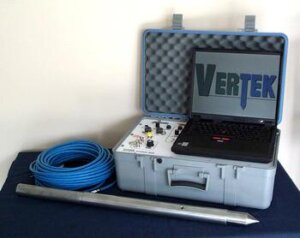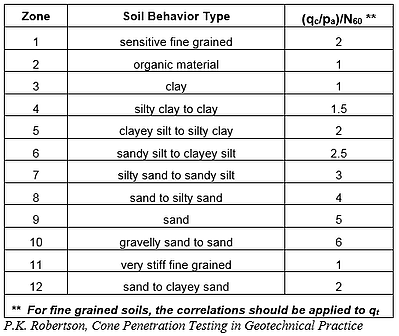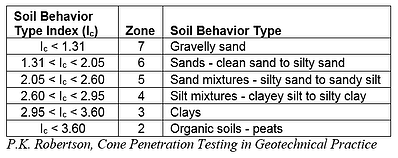As you know, Cone Penetration Testing is not the only method for determining the mechanical properties of soil. Another method is the Standard Penetration Test, or SPT: in this test, a borehole is drilled to a desired depth, then a hollow sampler is inserted and driven downwards with a hammer. The hammer blows are counted until the sampler travels the desired depth (usually 18”) – this number, denoted NSPT, indicates the mechanical properties of the soil. As with CPT data, a handful of corrections are commonly applied: for example, the N60 value indicates NSPT data corrected for the mechanical efficiency of a manual hammer, estimated at 60% at shallow overburden conditions.
Since SPT is one of the most common in-situ soil testing methods, you may find it necessary to compare information from both SPT and CPT tests, or convert from one set of parameters to the other, for example from SPT N60 values to CPT tip resistance values. Several methods have been proposed for calculating this relationship. Below are two of the most frequently used:

• Robertson and Campanella:

This method for correlating SPT and CPT data uses the following relationship between SPT N60 data and CPT tip resistance:
(qc/pa)/N60

qc = tip resistance (psi)
pa = atmospheric pressure (psi)
Soil behavior type can be determined from this equation based on the following table:This is perhaps the simplest method for relating the results of these two tests, but it can cause some confusion when the results fall on the border of two soil behavior type zones, or in situations where the ratio of CPT to SPT data could indicate one of several different soil types.

• Jefferies and Davies:

This is a more robust method for determining SPT N-values based on CPT data, or vice versa. It avoids the discontinuities of the first method, but it is not applicable to soil behavior type zones 1 (sensitive fine grained soils), 8 (very stiff sand to clayey sand), and 9 (very stiff fine grained soils).
First, one calculates the Soil Behavior Type Index, Ic, from the CPT data:
Ic = ((3.47 – log Qt)2 + (log Fr + 1.22)2)½
Qt = normalized tip resistance (dimensionless)
Fr = normalized friction ratio (%)
Next, the following equation relates the soil behavior type index to the SPT N60 value:
(qc/pa)/N60 = 8.5(1 – Ic/4.6)
Using these equations, one can easily work back and forth from one set of parameters to the other, or estimate soil behavior type directly from the calculation of Ic, per the table below.